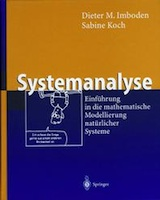Mathematically modeling natural systems

Systems and their mathematical description play an important role in all branches of science. This book offers an introduction to mathematical modeling techniques. It's intended for undergrad students in applied natural science, in particular earth and environmental science, environmental engineering, as well as ecology, environmental chemistry, chemical engineering, agronomy, and forestry.

What the book is

Step by step, we'll learn how to build models using basic mathematical components. While the intention is for you to build models yourself, the skills you learn will also be useful to analyze the properties of existing models. To support independent learners, numerous examples and problems from various scientific disciplines are provided throughout the book.

What the book isn't

The book doesn't derive mathematical formulas (some necessary mathematical knowledge is summarized in the appendix). That's not to say that there's no math in the book: to really understand what goes on inside a model we do need some math. The book also doesn't describe any modeling software (there are other books that do so).

What's on the website

You'll find the solutions to the problems in the book, and a list of other recommended books. Most importantly, we have provided several interactive examples for you to explore the models in a more practical, hands-on fashion:

The German versionThe book is based on a German original, which itself was based on a lecture called Systems Analysis held every year for undergraduate students in environmental sciences and geoscience at ETH Zürich. More details about this version can be found on the publisher's website.